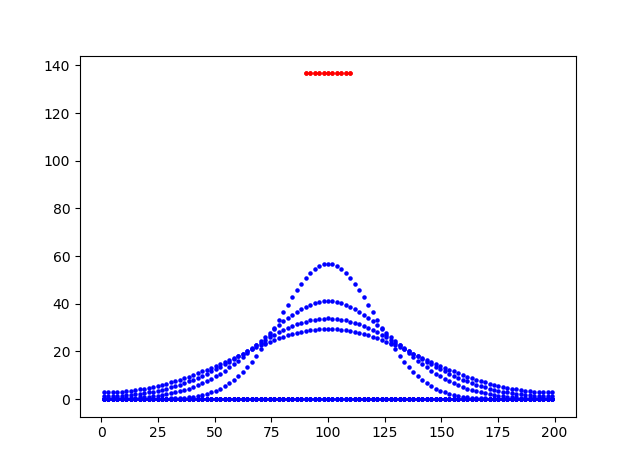## Different reaction rates in different sections

Extending NEURON to handle reaction-diffusion problems.

Moderators: hines, wwlytton, ramcdougal

RBJ
Posts: 56
Joined: Sun Aug 02, 2015 4:28 am

### Different reaction rates in different sections

Hello there,
I am trying to model the dynamics of a hypothetical factor (tfact) throughout a cell using RXD. To make what I believe to be the simplest possible model have made a two section cell, with 101 segments in each. I have linked them to one another. I added an "enzyme" rate reaction to one half (the right) not the other. I finally initialise with a high concentration of factor right in the middle and then watch the species travel through the cell. I expect to see that it spreads freely by diffusion into the left of the cell, but fails to get far into the right because of the rate reaction.
I don't get that, it seems my rate reaction is constant throughout this two-segment cell? (y=concentation, x =cell distance, initial red, blue is time-lapse snapshots, basically copied from the Tutorial on the Neuron website).Can anybody help? thank you.
The code I used in its entirety (sorry, I don't have a clue where I went wrong):

Code: Select all

``````from neuron import h, rxd
import numpy
from matplotlib import pyplot

# needed for standard run system

cellLEFT=h.Section()
cellRIGHT=h.Section()

cellLEFT.nseg=100
cellRIGHT.nseg=100
cellLEFT.connect(cellRIGHT(0))
#cellRIGHT.connect(cellMID(1))

tfact0=137
cytosol=rxd.Region([cellLEFT])
enzymerich=rxd.Region([cellRIGHT])
#d=diffusion constant
tfact = rxd.Species([cytosol,enzymerich],d=1, initial=0)

#start just with diffusion so blank these
reaction = rxd.Rate(tfact, 100000,regions=enzymerich)

h.finitialize()
for node in tfact.nodes:
if node.x > .45 and node.x < .55: node.concentration = tfact0
print('Nodes=',len(tfact.nodes.concentration))

def plot_it(color='b'):
y = tfact.nodes.concentration
x = tfact.nodes.x
# convert x from normalized position to microns
x = (cellLEFT.L+cellRIGHT.L) * numpy.array(x)
pyplot.scatter(x,y,marker='o',color=color,s=5)

#plot the initial situation
plot_it('r')

#We will plot every 25ms 5 up to 100ms
for i in range(1, 5):
#I presume this is h.continuerun(ms)
h.continuerun(i * 25)
plot_it()

pyplot.show()
``````

Posts: 38
Joined: Tue Apr 18, 2017 10:05 am

### Re: Different reaction rates in different sections

Here's a working version of your code:

Code: Select all

``````from neuron import h, rxd
from matplotlib import pyplot

# needed for standard run system

left = h.Section(name='left')
right = h.Section(name='right')

left.nseg = right.nseg = 101
left.L = right.L = 101

right.connect(left)

tfact0 = 137
cytosol = rxd.Region([left, right])
tfact = rxd.Species(cytosol, d=1, initial=0)

def right_only(node):
return 1 if node.x3d > left.L else 0

production_region = rxd.Parameter(cytosol, initial=right_only)
reaction = rxd.Rate(tfact, 0.2 * production_region)

h.finitialize(-65)
for node in tfact.nodes:
if 90 < node.x3d < 110:
node.concentration = tfact0

def plot_it(color='b'):
y = tfact.nodes.concentration
x = [node.x3d for node in tfact.nodes]
pyplot.scatter(x, y, marker='o', color=color, s=5)

# plot the initial situation
plot_it('r')

# we will plot every 25ms up to 100ms
for i in range(1, 5):
# run for another 25ms
h.continuerun(i * 25)
plot_it()

pyplot.show()
``````Explanation: There is no diffusion between different regions; to limit a Rate to a part of a region you can use an rxd.Parameter. When setting the initial values and plotting the results, remember node.x gives the relative position along a section. You can use node.x3d to give the spatial x location of the node. We also changed the connect here so that `right(0)` connects to `left(1)`.

(In the course of responding to your question, we found an unrelated bug in how Rate handles regions; this will be fixed in the next release. Thank you!)

We're planning on updating the reaction-diffusion tutorials. Do you mind if we use this model or something like it as an example?

RBJ
Posts: 56
Joined: Sun Aug 02, 2015 4:28 am

### Re: Different reaction rates in different sections

Thank you very much for your detailed help and speed! It will take a little while for me to process the information, but in the meantime please do use the code if it is useful. Kind Regards Richard

RBJ
Posts: 56
Joined: Sun Aug 02, 2015 4:28 am

### Re: Different reaction rates in different sections

Thank you again, so I have now "processed" that new information and help. I get it. In doing so I clocked that this is 1d, and that had been confusing me. I really want to get 3d. Having read your 2018 paper, Newton et al 2018, I realised that to get 3d I should at least be adding

Code: Select all

``rxd.set_solve_type(dimension=3).``
With the very latest windows binary installed this morning, and a fairly reasonable workstation this fails for me. There is no error thrown as such. It sits on the h.finitialize(-65) command for an hour or so. I will leave it running overnight (GMT), but I am assuming that a 1d task that took <30 seconds should not extend to beyond an hour even when 3d.
Also, if it helps, I thought to add the rxd.nthread(8) line as per Newton et al 2018, but that command is not recognised. I ploughed through the rxd python source code/module too and it doesn't seem to be in there. So is that a development feature (or did I miss it)?
kindest Regards
Richard
==STOP THE PRESS==
Sorry the 3D option did work on one of my PCs!!, it just took 4hrs to run.
R.